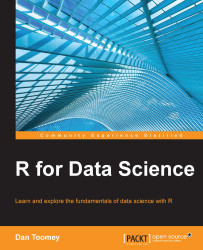•#### R for Data Science#### Overview of this book

R for Data ScienceCreditswww.PacktPub.comPrefaceFree Chapter
Data Mining PatternsData Mining SequencesText MiningData Analysis – Regression AnalysisData Analysis – CorrelationData Analysis – ClusteringData Visualization – R GraphicsData Visualization – PlottingData Visualization – 3DMachine Learning in ActionPredicting Events with Machine LearningSupervised and Unsupervised LearningIndex## Summary

In this chapter, we discussed different aspects of correlation using R. We determined the correlations between datasets using several methods and generated the corresponding graphics to display the correlation values. We were able to determine the correlations among binary data observations. Similarly, we computed the correlations between observations with a small number of responses. Lastly, we determined partial correlations.

In the next chapter, we will learn about clustering.